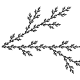3:44 AM
Would be suitable here instead of the deprecated tag; [Dimension of center of k[G]/rad k[G] when characteristic of k divides the order of G](mathoverflow.net/q/358050)? BTW the same question was posted yesterday on Mathematics: [Dimension of center of k[G]/rad k[G] where characteristic of k divides the order of G.](math.stackexchange.com/q/3632646)
1Let G be a finite group and consider k[G] where k is a field. In the scenario where char(k) divides |G|, how can one show that the dimension of Z(k[G]/rad k[G]) is strictly less than dimension of Z(k[G])? I'm trying to use this to show that in the case where char(k) divides |G|, the number of si...

3Let G be a finite group and consider k[G] where k is a field. In the scenario where char(k) divides |G|, how can one show that the dimension of Z(k[G]/rad k[G]) is strictly less than dimension of Z(k[G])? I'm trying to use this to show that in the case where char(k) divides |G|, the number of si...

2 hours later…6:09 AM
2Recently , I read one paper titled Modular equations and approximations to π by Ramanujan, in which there are some formulas for $q=\pi i \tau$( where $\tau=x+yi, y>0$, hence $|q|<1)$ : $$\prod_{n=1}^\infty\left(1+q^{2n-1}\right)=2^{\frac{1}{6}} q^{\frac{1}{24}}(kk')^{-\frac{1}{12}} ~~~ (1)$$ ...

1 hour later…7:15 AM
These queries should approximate list of tag creators. (It should show the first occurrence of the tag, but it does not find it if the question where the tag was created has already been deleted. At least for the recently created tags, most often this should be the user who created tag.)
Recently created tags: main, meta.
Most frequent tag creators: main, meta.
Recent edits in the tag-wikis/tag-excerpts are also useful information for people who care about tags.
Recent tag-info edits: main, meta.
Most frequent tag-info editors: main, meta.
As a side not, I should try to find time and check those tag-wikis which were plagiarized (without any attribution). Maybe most of them have been edited in the meantime.

1 hour later…8:30 AM
Perhaps the automated transformation would be less straightforward for links not to articles but to searches, authors, categories, etc. But probably there isn't too many of them here are a few examples. — Martin Sleziak 32 secs ago
Those are posts with links containing front.math.ucdavis.edu/search.
11I searched "Tower of Hanoi" on arXiv: The Group of Symmetries of the Tower of Hanoi Graph The Tower of Hanoi and Finite Automata Shortest paths in the Tower of Hanoi graph and finite automata Asymptotic aspects of Schreier graphs and Hanoi Towers groups A search on Wikipedia returned the Towe...

5I think you can get it from the Jacobi triple product identity $\prod_{m=1}^\infty (1-x^{2m})(1-x^{2m-1}y^2)(1+x^{2m-1}y^{-2}) = \sum_{n=-\infty}^\infty x^{n^2}y^{2n}$ We can set $x = q^{1/2}, y = q^{1/2}$. \[ \prod_{m=1}^\infty (1-q^{m})(1-q^{m+\frac{1}{2}})(1+q^{m-\frac{3}{2}}) = \sum_{n=...

2I'd say it's closer to an oriented manifold with corners (corners happening where the divisor is singular), or even that times a coefficient. In these papers Khesin, Rosly, and later Thomas build a homology theory based on this analogy.

16I understand the OP is frustrated with tight job market and those are valid concerns, but his/her description of the geometric analysis as a shallow subject is ridiculous. Parts of geometric analysis certainly attract top people and have seen remarkable recent progress. How a new Ph.D. could fai...

4In Dubuc and de la Vega's very nice write-up of Grothendieck's Galois theory they cover the pro-group case when the fibre functor is representable (see section 5.5): Consider a category $C$ and an object $A \in C$. Axioms on $C$: R1) $C$ has a terminal object and pullbacks (thus all fin...

9For details I recommend looking at the papers of Yagasaki on arxiv especially this paper. Let me answer 1. Consider be the diffeomorphism group of $\mathbb R^2$ that fixes $0$ and acts as the identity at the tangent space to $0$. The group acts on your space of embeddings by postcomposition. B...

3Don't need stable. It's the stack of all G bundles on a curve. The right hand side is the derived category of local systems, which are vector bundles with flat connection (G-bundles, for the Langlands dual of G in this equation). As for background, that depends on how deep an understanding you...

19One way to tell how active a field is is by looking at what's appearing on the arXiv in that area. I think that will show you that operator algebra is a robust subject with a lot of activity. In the comments, MaoWao points out that UC Berkeley and UCLA have very strong operator algebra groups, a...

0I'm currently studying with Melvyn Nathanson,who is really considered one of the experts on additive number theory.His texts,ADDITIVE NUMBER THEORY:THE CLASSICAL BASES and ADDITIVE NUMBER THEORY:INVERSE PROBLEMS are really the standard introductions to the subject.They are both published by Sprin...

1 hour later…9:35 AM
@MartinSleziak Now I noticed that MO has tag too. That one could be suitable for that question, too. mathoverflow.net/posts/358050/revisions

3 hours later…12:56 PM
I wanted to add a link to the paper cited in this question: Kazhdan Property T of semisimple Lie groups Google returns projecteuclid link - which is behind a paywall. And also this link.
I am not sure whether it should be added, as I am not familiar with the website "semanticscholar.org". (I do not know how reliable it is.)1:08 PM
A temporary link to get the list of linked questions updated: mathoverflow.net/questions/14311/…Martin Sleziak 56 secs ago
A temporary comment in order to get the list of linked questions updated: mathoverflow.net/questions/14311/…Martin Sleziak 6 secs ago

10 hours later…11:15 PM
2Let $G$ be a finite group and consider $k[G]$ where $k$ is a field. In the scenario where $\mathrm{char}(k)$ divides $|G|$, how can one show that the dimension of $Z(k[G]/\operatorname{rad}k[G])$ is strictly less than dimension of $Z(k[G])$? I'm trying to use this to show that in the case where ...